# Boolean Algebra – Introduction and Rules

The Boolean algebra works only with two values: 1/0, Yes/No, High/Low, H/L, True/False, On/Off. The possible options are those that respond to the logic of a switch. (On or Off)

When we design a digital circuit, it is common that at the end, we have a circuit with more than necessary number of gates. In order that the circuit has the least possible number of gates, we have to optimize it. When the digital circuit has been optimized, it will be:

– The simplest
– The number of components is the lowest
– The price of the project will be the lowest
– The power consumption of the circuit will be the lesser
– Maintenance of the circuit will be the easiest.
– The necessary space to implement the circuit is the lower. (on the PCB)

In summary, the design will be the cheapest possible.

A tool to reduce logical expressions of digital circuits. It is the mathematics of logical expressions, which was presented by George Boole in 1854. This tool is known as Boolean algebra.

Important notes: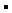(Dot) means logical product + (Plus sign) means logical addition

## The rules of Boolean algebra are:

Basic operations in Boolean algebra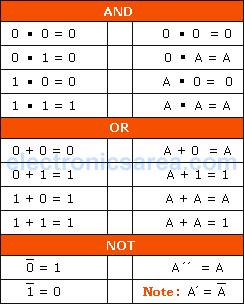Distributive Law, Associative Law, Commutative Law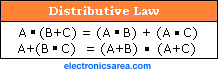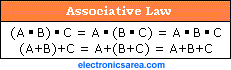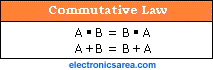Precedence and the Morgan Theorem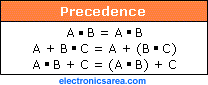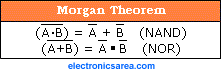To ensure that the reduction of the digital electronic circuit was successful, you can use the truth table. The truth table should give the same result for both, the original and to the simplified circuit.

•
•
•
•
•
•
•
•
•
•
•
•
•
•
•
•
•
•